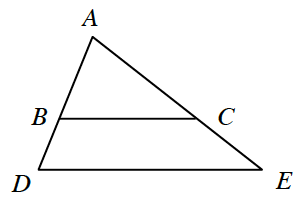### Home > GC > Chapter 5 > Lesson 5.3.2 > Problem5-82

5-82.In the diagram at right, $ΔABC$ and $ΔADE$ are similar. If $AB=5$, $BD=4$, and $BC=7$, then what is $DE$?

Separate the triangles into two similar triangles.

What is the length of $AD$? Once you know this, since the triangles are similar, the ratios of corresponding sides are equal.
Remember to set up proportions.

$DE=12.6$ units